Importance: High ✭✭✭
 Author(s): Ahmed, Tanbir Snevily, Hunter S.
 Subject: Combinatorics
 Keywords:
 Posted by: Tanbir Ahmed on: October 14th, 2013

Letdenote the set of all permutations of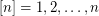. Letand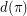denote respectively the number of increasing and the number of decreasing sequences of contiguous numbers in. Let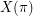denote the set of subsequences ofwith length at least three. Letdenote.

A permutation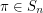is called a Roller Coaster permutation if. Let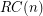be the set of all Roller Coaster permutations in.

Conjecture   For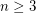,
\item If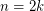, then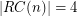. \item If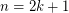, then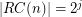with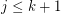.
Conjecture  (Odd Sum conjecture)   Given,
\item If, thenis odd for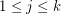. \item If, then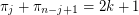for all.

## Bibliography

*[AS] Tanbir Ahmed, Hunter Snevily, Some properties of Roller Coaster permutations. To appear in Bull. Institute of Combinatorics and its Applications, 2013.

* indicates original appearance(s) of problem.